Journal topic
Wind Energ. Sci., 4, 595–618, 2019
https://doi.org/10.5194/wes-4-595-2019Wind Energ. Sci., 4, 595–618, 2019
https://doi.org/10.5194/wes-4-595-2019Research article 11 Nov 2019

Research article | 11 Nov 2019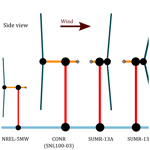# System-level design studies for large rotors

System-level design studies for large rotors
Daniel S. Zalkind1, Gavin K. Ananda2, Mayank Chetan3, Dana P. Martin4, Christopher J. Bay5, Kathryn E. Johnson4,5, Eric Loth6, D. Todd Griffith3, Michael S. Selig2, and Lucy Y. Pao1 Daniel S. Zalkind et al.
• 1Department of Electrical, Computer & Energy Engineering, University of Colorado Boulder, Boulder, CO 80309, USA
• 2Department of Aerospace Engineering, University of Illinois Urbana-Champaign, Champaign, IL 61820, USA
• 3Department of Mechanical Engineering, University of Texas at Dallas, Richardson, TX 75080, USA
• 4Department of Electrical Engineering, Colorado School of Mines, Golden, CO 80401, USA
• 5National Wind Technology Center, National Renewable Energy Laboratory, Golden, CO 80401, USA
• 6Department of Mechanical and Aerospace Engineering, University of Virginia, Charlottesville, VA 22904, USA

Correspondence: Daniel S. Zalkind (dan.zalkind@gmail.com)

Abstract

We examine the effect of rotor design choices on the power capture and structural loading of each major wind turbine component. A harmonic model for structural loading is derived from simulations using the National Renewable Energy Laboratory (NREL) aeroelastic code FAST to reduce computational expense while evaluating design trade-offs for rotors with radii greater than 100 m. Design studies are performed, which focus on blade aerodynamic and structural parameters as well as different hub configurations and nacelle placements atop the tower. The effects of tower design and closed-loop control are also analyzed. Design loads are calculated according to the IEC design standards and used to create a mapping from the harmonic model of the loads and quantify the uncertainty of the transformation.

1 Introduction

Wind turbines are large, dynamic structures that experience significant structural loading on their component parts. Design choices impact the loading on each of these parts. We present a model for the rapid computation of wind turbine design loads, which we use to quantify the effect of design trade-offs associated with different rotor concepts. The economics of wind energy have enabled larger wind turbine sizes, generator ratings, and blade lengths. Longer blades are economical simply because they capture more power more often. A wind turbine's annual energy production (AEP) is the total amount of energy captured by a wind turbine during one year. Increasing the power capture is the primary driver of reducing the cost of wind energy (COE)

$\begin{array}{}\text{(1)}& \mathrm{COE}=\frac{\mathrm{CapEx}+\mathrm{OpEx}}{\mathrm{AEP}},\end{array}$

where capital expenditures (CapEx) and operational expenditures (OpEx) make up the cost of building and running a wind turbine. Our goal is to minimize the cost of wind energy, enabling the sale of more wind turbines in an effort to make low-cost energy more available.

Operational expenditures are non-negligible but make up roughly 15 % of the total cost, according to a study of the average 2015 offshore wind turbine . Capital expenditures include the wind turbine parts and balance-of-station costs. Balance-of-station costs account for about 55 % of the total cost and include electrical infrastructure, assembly, and substructure costs. Wind turbine parts (tower, nacelle, blades, etc.) comprise about 30 % of the overall cost of an offshore, fixed-bottom wind plant . The small cost contribution of the wind turbine blades, which is only a fraction of the cost of the wind turbine parts, and the significant effect of wind turbine blades on AEP contribute to the economics that enable larger and larger blades.

However, longer blades require additional structural reinforcement, which increases the blade weight, resulting in larger loads experienced by other wind turbine components like the hub, main bearing, yaw bearing, and tower. Various innovations have enabled lower weight blades; these innovations are then used to subsequently design larger blades that capture more power. Still, the wind turbine components must survive extreme structural loading and last 20–30 years. Wind turbine components are often designed by various engineering teams based on loads from aeroelastic simulations, making wind turbine design a large, distributed design task.

The aerodynamic and structural aspects of wind turbines must be designed and controlled so that the structural loading for a design is feasible. There is a large interdependence between these design aspects (aerodynamic, structural, and controls) and on the various wind turbine components, which has led to numerous design optimization studies. These studies focus primarily on blade aerodynamic and structural design, e.g., in and . Some incorporate dynamic control effects, like and . System engineering tools, like HAWTOpt2 (Døssing2011), WISDEM , and Cp-Max , have been developed to handle the large number of design variables but often compute structural loads using simplified scaling rules, conservative static calculations, or many nonlinear aeroelastic simulations. A full set of design load cases (DLCs), specified by the (IEC) in design standards, and simplified for research purposes in , can include up to 2000 simulations, which can be costly in terms of computational effort, resulting in long design cycle times. Often the results of these simulations do not fully elucidate the root cause of problematic load cases on the affected turbine component. An attempt to distill the DLCs into a reduced basis for design loads in an optimization framework was presented in .

The power and load estimation procedure developed in this study is used to analyze concepts for enabling rotor radii greater than 100 m. Recently, large rotor concepts have been studied in the European projects UpWind and INNWIND. The Danish Technical University (DTU) 10 MW reference wind turbine (RWT) was provided as a design basis for large rotors to test design methods and tools. The DTU 10 MW RWT has motivated studies that focus on optimization methods and active and passive load control methods, but the resulting designs from these studies do not deviate far from the base rotor model. A two-bladed, downwind, teetering hub configuration of the DTU 10 MW RWT was developed, which shows that a teetering hub can greatly reduce the unbalanced loading on the main shaft and blade root . suggest that the tower stiffness distribution needs to be redesigned in order to avoid a resonance at the twice-per-revolution (2P) rotor harmonic and that two-bladed rotors (without teeter) increase loading on the main shaft significantly.

A couple of 20 MW rotor designs have been proposed in the literature. and use classical similarity scaling rules to upscale conventional turbines. Both conclude that loads due to self-weight will increase significantly with blade length and drive component design as turbines grow larger. Specifically, edgewise blade loads and the effect of wind shear are magnified for larger rotor sizes.

A series of design studies at Sandia National Laboratories (SNL) detailed the structural design of a 100 m blade with the goal of reducing the blade mass. First, a classically upscaled blade was given a detailed composite lay-up and tested against DLCs . Next, a series of design innovations reduced the blade mass from 76 metric tons to 49 metric tons, utilizing carbon-fiber reinforcement , advanced core materials , and flatback airfoils .

Another concept to reduce mass-scaling issues is a highly coned, downwind rotor, which has shown that blade loads can be reduced by converting large cantilever loads at the blade root into tensile loads along the span of the blade . We will analyze this concept and its effect on the structural loading of the other wind turbine components besides the blades.

There are few openly published documents that quantify the effects of significant design changes and detailed rotor upscaling on the various wind turbine components. We will quantify the effect of aerodynamic changes, including the blade length, axial induction, cone angle, and number of blades, as applied to both upwind and downwind rotors. A simplified structural model will demonstrate the effect of structural reinforcement on blade mass and loads. The upscaled structural model must provide enough stiffness to compensate for the increasing edgewise blade loads of large rotors. We quantify the effect of changes to the hub by looking at three-bladed and two-bladed rotor configurations, and consider the relative benefits of a teeter hinge or individual pitch control for the latter. Finally, we show how the nacelle placement atop the tower and control schemes can impact the loads on the tower and yaw bearing.

We will present the baseline models used for comparison and our general design direction in Sect. 2. Section 3 will outline the tools used for design and simulation and will also provide environmental site specifics. A description of the control scheme used throughout the article is presented in Sect. 4. The harmonic model is described in Sect. 5, and in Sect. 6 the transformation from harmonic loads to DLC-simulated design loads is described. The set of design studies is described in Sect. 7, leading to studies of blade loads and power capture (Sect. 8), hub and main-bearing loads (Sect. 9), yaw-bearing loads (Sect. 10), and tower loads (Sect. 11). A discussion of the model's limitations and potential use is provided in Sect. 12, followed by conclusions in Sect. 13.

2 Baseline models and design direction

It is useful to start from established designs when doing comparative analysis. In Sect. 8.2, in lieu of a full structural lay-up design, we will use these baseline models for scaling the distributed structural properties of rotor blades. For three-bladed rotors, we will use a conventional rotor design (CONR-13) as a starting point. The CONR-13 is the culmination of a series of design studies aimed at designing a lightweight 100 m blade; it utilizes flatback airfoils, carbon-fiber reinforcement, and advanced core materials to reduce the blade mass below state-of-the-art scaling trends. The full design is described in . The distributed blade structural properties of the CONR-13 will be used for all three-bladed rotors in this study.

A downwind, two-bladed rotor was developed with similar structural advances but with the goal of reducing the total blade mass by at least 25 % compared to the CONR-13 (Griffith2017). The blade was designed to enable segmentation, ultralight design, and a morphing rotor; we refer to this design as the SUMR-13A. The initial aerodynamic design is presented in . We have slightly modified the initial design to have a downwind cone angle of 5 for the purposes of the design studies presented later. The distributed structural parameters of the SUMR-13A blade were used as a basis for scaling all two-bladed rotors in this study. A summary of both baseline models is shown in Table 1 and are drawn to scale in Fig. 1. Both rotors were structurally validated to check strain limits, panel buckling, flutter, and fatigue.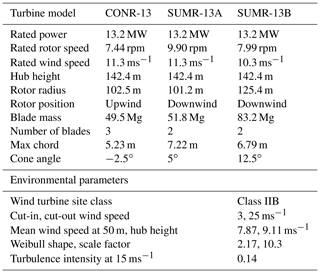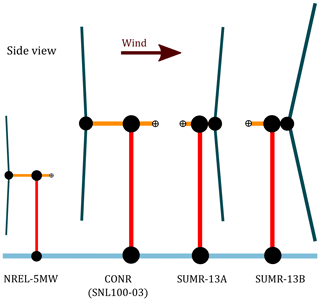Figure 1Illustrations of the turbines in this study, along with the National Renewable Energy Laboratory (NREL) 5 MW reference turbine for comparison. Tower heights, rotor radii, and cone angles are drawn to scale; overhangs and nacelle center of masses are enlarged for comparison.

In the remainder of this paper, we will evaluate designs aimed at

1. increasing the energy capture and

2. reducing the wind turbine component loads.

To reduce the cost of energy in Eq. (1), it is most important to increase energy capture (AEP). Industry trends suggest a continued increase in blade length, leading to greater loads on all turbine components. Structural loads contribute to component design and capital cost (CapEx) but require detailed design and cost models for each individual part. Instead of a detailed cost analysis, which is specific to the component supplier and subject to uncertainty, we will develop a larger rotor design, called the SUMR-13B, described in Sect. 8.1, and then quantify the changes to global wind turbine loads and power capture while exploring techniques to reduce those loads.

3 Design and simulation tools, wind turbine environment

Aerodynamic design was performed using two inverse design tools: PROPID and PROFOIL. PROPID is an inverse rotor design tool that enables a rotor geometry to be designed based on desired performance specifications like available power, tip speed ratio, wind speed distribution, axial induction, airfoils used, and desired lift distribution along the blade. PROFOIL is an inverse airfoil design tool. It allows for the design of airfoil geometries based on prescribed velocity distributions and desired geometric (thickness and camber) and aerodynamic properties. Airfoil geometries output using PROFOIL are analyzed using XFOIL (Drela1989) and iterated on using PROFOIL until a final converged design is obtained.

Aeroelastic simulations were performed using the latest version of FAST (Jonkman2013). Different FAST modules couple the wind inflow with aerodynamic and elastic solvers that compute the structural loading on the wind turbine. Turbulent wind inputs are generated using TurbSim . A recent FAST-based, wind-tunnel-validated approach has shown that, compared with turbulence, tower shadow effects are relatively small . Thus, for simplicity, we have omitted the tower shadow model from our analysis in order to focus on the influence of the more important harmonic and turbulent loads. Control inputs are provided to FAST through a Matlab/Simulink interface that processes FAST outputs and performs closed-loop control. Fatigue results are computed using MLife (Hayman2012), which uses a rain-flow-counting algorithm to determine load cycles and extrapolates them over the lifetime of the wind turbine.

To properly compute lifetime fatigue and annual energy production, the wind turbine environment must be provided. The rotors in this study are all designed to be placed off the coast of Virginia, USA. The site corresponds to a Class IIB turbine rating , with mean and turbulent wind speed characteristics shown in Table 1.

4 Closed-loop control

To simulate turbine design loads and power capture, a closed-loop control scheme is necessary. In below-rated conditions, the generator torque τg is controlled so that the rotor speed ω is optimal for power capture, following the typical τg=kω2 law for most of the below-rated operating region, before transitioning to above-rated conditions . For simplicity, this is implemented as a look-up table, though more sophisticated methods exist. The look-up table is altered to avoid a critical rotor speed for two-bladed rotors only (see Fig. 2b; Sect. 11 provides more details). The generator rated power of 13.2 MW and rated speed of 1173.7 rpm are assumed to be constant for all the turbines in this study. The gearbox ratio of each turbine is changed to enable operation at the aerodynamically optimal rated rotor speed.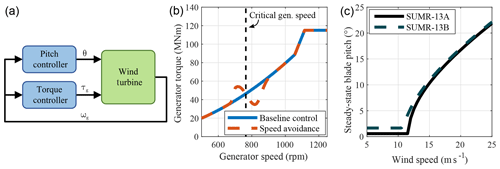Figure 2Baseline control block diagram, where θ is the pitch angle, τg is the generator torque, and ωg is the measured generator speed (a). The torque control signal (b) for baseline control (blue) and speed avoidance control (red) to avoid the critical generator speed. Steady-state blade pitch angles (c) for the SUMR-13A and SUMR-13B.

In above-rated wind speeds, the pitch angle is controlled to regulate the rotor speed to its rated value using a gain-scheduled proportional-integral (PI) controller. The gains of the PI controller are set so blade fatigue is minimized, subject to a constraint on the maximum generator speed . We have chosen this control architecture, which is the same for all rotors, so that it can be easily tuned for many rotors in the same way. The optimal generator torque control gain k is computed using rotor parameters, and the PI pitch control gains are tuned using a subset of the DLC 1.2 turbulent simulations. The control architecture (as shown in Fig. 2a) is adapted from the National Renewable Energy Laboratory (NREL) 5 MW baseline controller , which is commonly used as a reference to compare new controller designs. While this baseline control may not necessarily be the best possible controller, it allows us to focus on the power and load sensitivity to model changes.

Using closed-loop control for load simulations is important because peak loads often occur near the transition between below- and above-rated operation. With a constant generator rating (13.2 MW), different rotors transition from below- to above-rated conditions at different wind speeds. Additional control signals, like individual pitch control (IPC) signals, are added to the baseline control signals in Fig. 2a.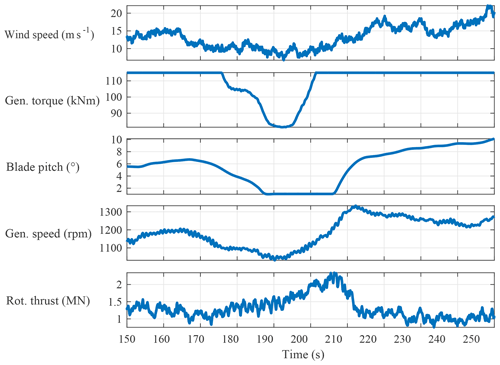Figure 3Baseline control illustration of a problematic gust for the SUMR-13A baseline rotor in extreme turbulence (DLC 1.3) with a mean wind speed of 14 ms−1. The peak rotor thrust near 205 s causes the peak blade flapwise load for the SUMR-13A.

A controller is also necessary for computing design loads in turbulent DLC simulations, where wind speed changes, or gusts, must be adequately controlled. Often, peak loads are caused by a negative gust, or lull, which we show in Fig. 3. During a decrease in wind speed, the rotor slows and the pitch decreases to its optimal power position. When the decrease in wind speed is followed by a positive gust, the pitch control must react quickly to regulate rotor speed. We model the actuator of each rotor in this study as a second-order Butterworth filter with a cut-off frequency of 0.25 Hz. The pitch actuator has a maximum pitch rate limit of 4 s−1; maximum pitch rates between 1 and 3 s−1 were recorded in the turbulent simulations that were run. This decrease and then increase in wind speed creates a condition where there is an above-rated wind speed but a below-rated pitch angle setting, resulting in a large thrust force on the rotor and high loads. To capture the effect that closed-loop control has on design loads as rotor changes are made, we use the same control architecture for computing loads using the harmonic model (Sect. 5) and for turbulent DLC simulations (Sect. 6), updating the controller parameters based on the rotor parameters.

5 Harmonic model for load estimation

The harmonic loads are derived from FAST simulations with a sheared wind inflow such that the wind speed u at height z is

$\begin{array}{}\text{(2)}& u\left(z\right)={u}_{\mathrm{h}}{\left(\frac{z}{{z}_{\mathrm{h}}}\right)}^{\mathit{\alpha }},\end{array}$

where zh is the hub height, uh is the wind speed at hub height, and α=0.14, which is representative of an offshore wind field . Because of the wind shear, the turbine's structural load signals contain harmonic components that depend on the rotor azimuth ψ; i.e., a load signal m(ψ) can be expressed as

$\begin{array}{}\text{(3)}& \begin{array}{rl}m\left(\mathit{\psi }\right)& ={m}_{\mathrm{0}}+{m}_{\mathrm{c}}^{\mathrm{1}\mathrm{P}}\mathrm{cos}\left(\mathit{\psi }\right)+{m}_{\mathrm{s}}^{\mathrm{1}\mathrm{P}}\mathrm{sin}\left(\mathit{\psi }\right)+\phantom{\rule{0.125em}{0ex}}\mathrm{\dots }\\ & +{m}_{\mathrm{c}}^{i\mathrm{P}}\mathrm{cos}\left(i\mathit{\psi }\right)+{m}_{\mathrm{s}}^{i\mathrm{P}}\mathrm{sin}\left(i\mathit{\psi }\right)+\phantom{\rule{0.125em}{0ex}}\mathrm{\dots }\end{array}\end{array}$

The components are computed by

$\begin{array}{}\text{(4)}& & {m}_{\mathrm{0}}=\frac{\mathrm{1}}{\mathrm{2}\mathit{\pi }{N}_{\mathrm{R}}}\underset{\mathit{\psi }-\mathrm{2}\mathit{\pi }{N}_{\mathrm{R}}}{\overset{\mathit{\psi }}{\int }}m\left(\mathit{\psi }\right)\mathrm{d}\mathit{\psi },\text{(5)}& & {m}_{\mathrm{c}}^{i\mathrm{P}}=\frac{\mathrm{1}}{\mathit{\pi }{N}_{\mathrm{R}}}\underset{\mathit{\psi }-\mathrm{2}\mathit{\pi }{N}_{\mathrm{R}}}{\overset{\mathit{\psi }}{\int }}m\left(\mathit{\psi }\right)\mathrm{cos}\left(i\mathit{\psi }\right)\mathrm{d}\mathit{\psi },\end{array}$

and

$\begin{array}{}\text{(6)}& {m}_{\mathrm{s}}^{i\mathrm{P}}=\frac{\mathrm{1}}{\mathit{\pi }{N}_{\mathrm{R}}}\underset{\mathit{\psi }-\mathrm{2}\mathit{\pi }{N}_{\mathrm{R}}}{\overset{\mathit{\psi }}{\int }}m\left(\mathit{\psi }\right)\mathrm{sin}\left(i\mathit{\psi }\right)\mathrm{d}\mathit{\psi },\end{array}$

where NR is the number of rotations used in the calculation . We have found that load signals can be reconstructed closely using the first four harmonics; the most energy is usually in either the first, second, or third harmonic depending on the component (see Table 2) and number of blades.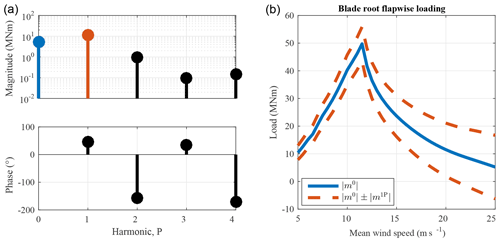Figure 4Load harmonic magnitude $|{m}^{i\mathrm{P}}|$ and phase ϕiP for the zeroth through fourth periodic harmonic of the blade root load in the flapwise direction (a) of the SUMR-13A at 25 ms−1. Mean load (blue) superimposed with the 1P harmonic amplitude (red) with respect to wind speed (b) used to estimate fatigue and extreme loads.

From the components in Eqs. (5) and (6), the magnitude and phase of each harmonic can be computed:

$\begin{array}{}\text{(7)}& \left|{m}^{i\mathrm{P}}\right|=\sqrt{{\left({m}_{\mathrm{c}}^{i\mathrm{P}}\right)}^{\mathrm{2}}+{\left({m}_{\mathrm{s}}^{i\mathrm{P}}\right)}^{\mathrm{2}}},\end{array}$

and

$\begin{array}{}\text{(8)}& {\mathit{\varphi }}^{i\mathrm{P}}={\mathrm{tan}}^{-\mathrm{1}}\left(\frac{{m}_{\mathrm{s}}^{i\mathrm{P}}}{{m}_{\mathrm{c}}^{i\mathrm{P}}}\right).\end{array}$

An example for the blade flapwise load is shown in Fig. 4; most of the load magnitude is in the constant m0 and once-per-revolution m1P load component (101–102 MNm), with some in the 2P load component due to shaft tilt and gravity (∼100 MNm), and very little in the higher harmonics ($<{\mathrm{10}}^{-\mathrm{1}}$ MNm). We will use these harmonic coefficients, calculated via Eqs. (4)–(8), to estimate fatigue and extreme loads for the various wind turbine components.

## 5.1 Extreme and fatigue loads

The forces and moments on a component drive its design: larger loads require greater reinforcement, leading to greater component mass and cost. We analyze component loads in terms of the maximum (or peak) load:

$\begin{array}{}\text{(9)}& {m}_{\mathrm{Peak}}^{\mathrm{H}}={\mathrm{max}}_{u\in U}\left({m}^{\mathrm{0}}+{m}^{n\mathrm{P}}\right),\end{array}$

where n is the dominant harmonic signal component and U is the set of constant, sheared wind inputs used to derive the harmonic load. We perform simulations from cut-in to cut-out (Table 1) in 0.5 ms−1 increments.

Table 2Structural loads evaluated in this article. Each component has loads in multiple directions and experiences the peak load and greatest contribution to fatigue loads at different wind speeds. NB denotes the number of blades on the rotor. Loads that are nearly constant across wind speeds do not have a defined peak wind speed (N/A). The dominant wind speed contributing to fatigue is determined by analyzing the relative fatigue contribution, p(u)mnP from Eq. (10), across wind speeds.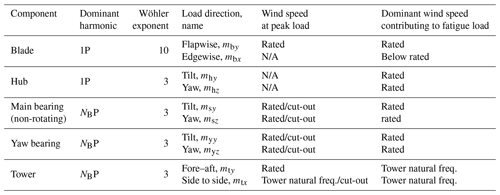N/A indicates “not applicable”.

Fatigue loads are computed in terms of the damage equivalent load (DEL): the constant amplitude of a sinusoidal load signal that results in the same total accumulated damage from a more complex load signal. The accumulated damage in simulations with different wind speeds is extrapolated over the turbine lifetime using the wind speed probability distribution p(u), characterized by the Weibull distribution in Table 1. We can relate the DEL of a component to its load harmonic by

$\begin{array}{}\text{(10)}& {m}_{\mathrm{DEL}}^{\mathrm{H}}={a}_{\mathrm{DEL}}\left(n,w\right)\sum _{u\in U}p\left(u\right){m}^{n\mathrm{P}},\end{array}$

where aDEL is a tuning factor that depends on the Wöhler exponent w and the dominant harmonic component n. The dominant load harmonic nP of each component is either 1P or NBP, specified in Table 2, depending on whether the component is rotating (1P) or non-rotating (NBP). Different load harmonics will be specified by their location, direction, and harmonic number; e.g., the 3P main-bearing load about the ys axis will be written ${m}_{\mathrm{s}y}^{\mathrm{3}\mathrm{P}}$. In this article, we focus on the moments about the load axes specified in Table 2 and illustrated in Fig. 5. The loads at higher harmonic and natural frequencies contribute to both fatigue and extreme loads, but since our goal is to derive a mapping from a simplified computation (harmonic load) to a more expensive simulation (design load), their effects are neglected and considered as part of the uncertainty of the transformation in Sect. 6.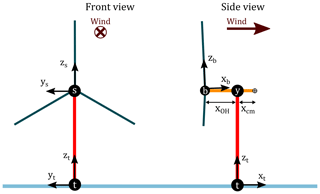Figure 5Illustration of the load axes used in this article. The non-rotating load axes – tower, main bearing, and yaw bearing – are all parallel and are denoted by subscripts “t”, “s”, and “y”, respectively. Note: the blade, hub, and main-bearing axis origins are collocated; the blade and hub load axes rotate with azimuth angle, as shown in Fig. 12. The CONR-13 is depicted to illustrate the rotor overhang xOH and nacelle center of mass xcm. The prevailing wind is positive in the same direction as the xt axis.

## 5.2 Harmonic versus turbulent loads

The structural loads on a wind turbine originate from constant and periodic effects, modeled by the harmonic load, as well as from dynamics due to turbulence, which are not necessarily correlated with the azimuthal position of the rotor and are not modeled in this transformation. In some cases, the effect of turbulence greatly outweighs the constant and periodic effects, but in all cases, the harmonic loads can be mapped to the design loads determined by the DLCs. We quantify this relationship in Sect. 6 by mapping the harmonic loads, computed using Eqs. (9) and (10), to the design loads computed in DLC simulations. In Sects. 711, we present the design load estimates and their uncertainties, transformed from harmonic loads, as various turbine design choices are evaluated.

6 Harmonic model transformation and uncertainty

To balance the computational efficiency of the harmonic load estimation in Sect. 5 with the more expensive and realistic design loads computed using DLC simulations, we present the following transformation procedure. In this article, we focus on the moments on the turbine components during power-producing design load cases and simulate the following DLCs specified by the IEC standard :

• DLC 1.2: normal turbulence, for fatigue loads, using six random seeds at mean wind speeds from cut-in to cut-out, spaced 2 ms−1 apart.

• DLC 1.3: extreme turbulence, for peak loads, using the same number of turbulent wind seeds and wind speeds.

• DLC 1.4: extreme coherent gust with direction change, for peak loads near rated, above-, and below-rated wind conditions. Different rotor azimuthal initial conditions are simulated to account for the rotor being in different positions when the gust occurs.

• DLC 1.5: extreme wind shear, for peak loads near rated and at cut-out wind speeds. The same azimuthal initial conditions as in DLC 1.4 are used.

Fatigue loads are computed using the DLC 1.2 simulations in MLife (Hayman2012); they are extrapolated using the Weibull distribution in Table 1 to determine the lifetime DEL. The peak design load is determined using the maximum (moment) over all the simulations in DLCs 1.3–1.5.

First, we compare the harmonic loads, calculated using the methods in Sect. 5, with the loads computed in DLC simulations. Then, we present a method to map the harmonic loads to the design loads, producing load estimates. Finally, we analyze the residual of the estimated loads, since not all rotors in the design studies of Sects. 711 will be simulated using the DLCs. Only a subset of the rotors analyzed in this article, indicated in Table 3, are used in the following procedure to transform the harmonic model. The design loads of a free-teetering hinge will not be included in the transformation set and uncertainty analysis for reasons described in Sect. 9.2; it is marked with an “x” in Fig. 6.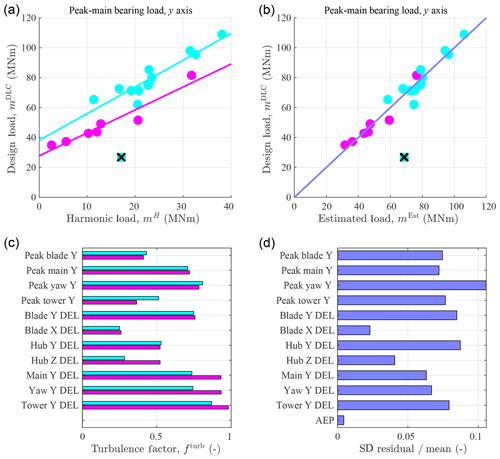Figure 6Peak main-bearing loads computed using DLC simulations versus the harmonic load (a) and transformed load estimates (b) for two-bladed rotors (cyan) and three-bladed rotors (magenta). The same color scheme is used to show the relative effect of turbulence on selected component loads (c), as defined in Eq. (12), and the standard deviation of the residual normalized by the mean load is shown for the whole transformation set (d). The loads presented in this study are specifically the moments about the specified axis.

$\begin{array}{}\text{(11)}& {m}^{\mathrm{DLC}}={m}^{\mathrm{H}}+{m}^{\mathrm{turb}}.\end{array}$

We quantify the turbulent load contribution mturb of each component load using the turbulence factor

$\begin{array}{}\text{(12)}& {f}^{\mathrm{turb}}=\frac{\mathrm{mean}\left({m}^{\mathrm{turb}}\right)}{\mathrm{mean}\left({m}^{\mathrm{DLC}}\right)}\end{array}$

to compare between different turbine parts on how much of the design load mDLC is attributed to turbulent versus harmonic loading for Class IIB turbulence.

We also see a difference in how turbulence affects two- versus three-bladed rotors, illustrated by the different lines of fit in Fig. 6a. In general, two-bladed rotors have a greater turbulent load component, but they also have a larger harmonic component, so the turbulence factor is similar to three-bladed rotors. For three-bladed rotors, the non-rotating load component DELs are not clearly modeled by their harmonic load, so they have a relatively high turbulence factor. Even though some turbine parts have large turbulent components that are not directly modeled by their harmonic loads, there is still good correlation between the harmonic and design loads.

We transform from the harmonic loads to the design loads by fitting a linear model,

$\begin{array}{}\text{(13)}& {m}^{\mathrm{DLC}}={a}^{\mathrm{trans}}{m}^{\mathrm{H}}+{b}^{\mathrm{trans}},\end{array}$

and finding the linear least squares estimate of the parameters atrans and btrans. Because two- and three-bladed rotors sample turbulence differently, we define a transformation set (atrans, btrans) separately for each, illustrated by the different fits of Fig. 6a. There are also different transformation sets for each design load: at each axis and for both peak and fatigue loads. To estimate the design load, the transformation set corresponding to the desired component, axis, and number of blades is used:

$\begin{array}{}\text{(14)}& {m}^{\mathrm{Est}}={a}^{\mathrm{trans}}{m}^{\mathrm{H}}+{b}^{\mathrm{trans}},\end{array}$

which results in a transformed load estimate equal to the design load, plus some residual (Fig. 6b).

We analyze the uncertainty of the transformation by computing the residuals between the estimated loads, which are fit using the linear relation (Eq. 14), and design loads of the set of rotors specified in Table 3. In Fig. 6d, we normalize the standard deviation of the residual by the mean load over all rotors to use a qualitative metric comparing the fit of the transformation across different turbine parts. We present the standard deviation of the residual without this normalization for each measure in the figures of Sects. 711.

In general, the standard deviation of the residual is less than 12 % of the mean value, which indicates decent agreement between the transformed load estimates and the DLC-computed design loads. The cases with lowest uncertainty tend to have lower turbulence factors, like the blade edgewise (blade X) DEL and the hub zh-axis DEL. The AEP is also very well estimated by the harmonic model, which is good for power capture predictions as long as the effects of turbulence are transformed.

The most erroneous load component is the peak yaw-bearing load about the yy axis, which has a large turbulent component and where a subset of the transformation set (the aerodynamic trade study designs) controls a problematic gust event, like the one in Fig. 3, similarly. These rotors have design loads that are about the same for each, despite the differences predicted by the harmonic model. The design loads for this component might be more a function of the gust event than the turbine configuration.

In the remainder of this article, we use these mapped load estimates to analyze the structural loading and power capture of the various rotor configurations in Table 3.

Table 3Set of turbines designed and analyzed in this article. a denotes a turbine for which DLC simulations were performed and used to map the harmonic load estimates to DLC-based design loads. Otherwise, only the harmonic load analysis is performed. b was omitted from the transformation set. c denotes the SUMR-13A rotor and d denotes a three-bladed variation of the SUMR-13A rotor. The process for using axial induction as an independent design variable will be described in the rotor aerodynamic trade studies section (Sect. 8.1).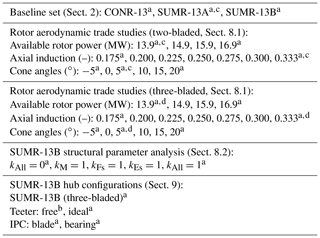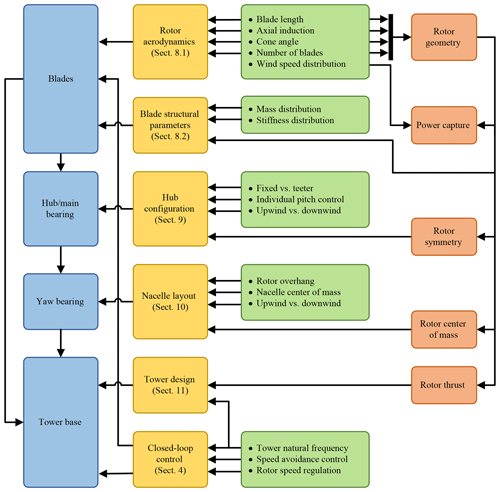Figure 7Overview of the design studies performed in this paper. The loads on each component (blue) transfer from the blades to the tower base as shown. Design studies (yellow) that affect each component are performed in Sects. 811 by altering the design parameters in green. Rotor design parameters (orange) affect all aspects of turbine design.

7 Overview of design studies

In this section, we outline the design and simulation results of the 42 turbines shown in Table 3. The design loads for each rotor are estimated using harmonic loads from Sect. 5 and the transformation method in Sect. 6. Additionally, gross AEP is calculated using the generator power P(u) at mean wind speed u by

$\begin{array}{}\text{(15)}& \mathrm{AEP}=\mathrm{8760}\sum _{u\in U}p\left(u\right)P\left(u\right),\end{array}$

where p(u) is the Weibull distribution in Table 1 and 8760 is the number of hours in a year.

## 8.1 Rotor aerodynamics

We evaluate rotors with longer blade lengths, lower axial induction factors, and large, downwind cone angles, using the SUMR-13A design described in Sect. 2 as a baseline. These design studies have led us to an updated, larger, two-bladed design, indicative of the trends in industry towards longer, more slender blades but with a greater downwind cone angle. We will call this new rotor SUMR-13B (see Table 1 for more details).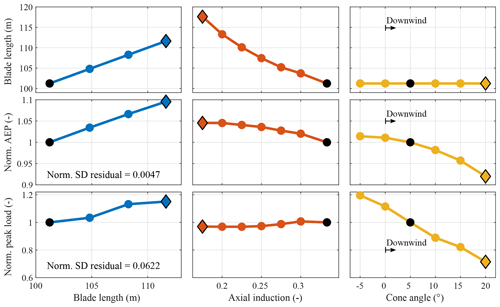Figure 8Summary of aerodynamic design studies: the blade length, axial induction (in combination with blade length, chord, and twist), and cone angle are varied, while the AEP and peak blade load are calculated and compared to the base case (SUMR-13A, black dot in all). The standard deviations of the residuals for AEP and peak flapwise load are normalized to the SUMR-13A values and apply across all design studies. All rotors here are two-bladed, and positive cone angles correspond to downwind rotors. Unless otherwise specified, the available rotor power is 13.9 MW, the axial induction is 0.333, and the cone angle is 5.

Blade length is changed indirectly in PROPID by increasing the available rotor power at 11.3 ms−1 from 13.9 to 16.9 MW. However, all rotors are controlled to have the same rated generator power of 13.2 MW, which limits the increase in peak blade loads by transitioning to above-rated control at lower wind speeds.1 The increased rotor-swept area increases both power capture and blade loads; a 10 % increase in rotor radius results in about a 10 % increase in AEP and 15 % increase in peak blade flapwise load (blue, left column in Fig. 8). For the blade length design study, the axial induction factor along the outer three-fourths of the blade is fixed at $\frac{\mathrm{1}}{\mathrm{3}}$ (theoretical Betz limit).

The rotors used to evaluate axial induction (red, center column in Fig. 8) are designed by fixing the flapwise root bending loads to that of the SUMR-13A and fixing the available rotor power at rated wind speed to 13.9 MW. The blade length, chord, and twist are allowed to vary as the local axial induction factor – from the 25 % radial location to the blade tip – varies from 0.175 to 0.3 in increments of 0.025. Decreasing the designed axial induction of the rotor results in longer, more slender blades that capture more energy while constraining blade loads. In the most extreme example, a blade with a 0.175 axial induction factor can increase the AEP by 5 %, compared to a rotor with aerodynamically optimal blades (axial induction factor of $\frac{\mathrm{1}}{\mathrm{3}}$) but requires 16 % longer blades.

The cone angle design study is performed using the same baseline SUMR-13A blades for each rotor but with different cone angles, including upwind (negative) and downwind (positive) cone angles. With a fixed blade length, downwind, highly coned rotors decrease the rotor-swept area, resulting in both reduced power capture and blade loads. The load decrease is significant: 25 % compared with a 7 % decrease in power capture. In comparison with the blade length design study, it is clear why highly coned rotors are attractive for large rotor designs: an increased cone angle will decrease operational loads faster than an increase in blade length will increase them.

For all the aerodynamic design studies, there is a trade-off between power capture and blade loading. Each design study is plotted together in Fig. 9, which also indicates the DELs in the flapwise and edgewise directions. In rotor design, our goal is to increase AEP and decrease blade loads, thus aiming to yield results in the lower right quadrant of each plot.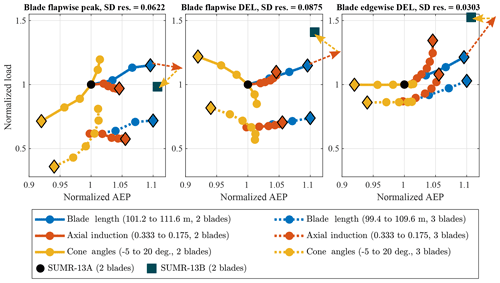Figure 9The trade-off between power capture and blade loads. The AEP is plotted on the x axis and blade loads are plotted on the y axis. All rotors are normalized to the two-bladed 101.2 m SUMR-13A baseline rotor design (black dot). Each dot represents a rotor design and each curve represents the variation of one design parameter. The set of three-bladed rotor designs is represented with dotted curves. Unless otherwise specified, the available rotor power is 13.9 MW, the axial induction is $\frac{\mathrm{1}}{\mathrm{3}}$, and the cone angle is 5; the SUMR-13B is specified in Table 1. The normalized residual standard deviation for AEP is the same as in Fig. 8, and the load residual standard deviations are normalized to the corresponding SUMR-13A values. The vectors indicate design changes in combination: blade length increase (blue diamond), axial induction factor decrease along with corresponding blade length increase (red, dashed vector), and cone angle increase (yellow, dashed vector) from the SUMR-13A to the SUMR-13B (square).

A set of three-bladed rotors (shown with dotted lines in Fig. 9) is designed similarly to the two-bladed design studies and exhibit similar trends to the two-bladed rotors in terms of blade loads. The blades of the three-bladed rotors experience lower loads (both peak and fatigue, edgewise and flapwise) with the same power capture due to their smaller chord and mass.

To model blades with different lengths, we start with classical similarity scaling rules , based on the length scaling factor:

$\begin{array}{}\text{(16)}& \mathit{\eta }=L/{L}_{\mathrm{0}},\end{array}$

where L is the length of the scaled blade and L0 is the length of the original blade. In this study, L0 is the length of the baseline blades: the SUMR-13A for two-bladed rotors and the CONR-13 for three-bladed rotors. We will examine the scaling of the following parameters :

• mass per unit length, which scales with η2;

• stiffness per unit length in the flapwise, edgewise, and torsional directions, which scales with η4;

• stiffness per unit length in the spanwise direction, which scales with η2; and

• inertia per unit length in the flapwise and edgewise directions, which scales with η4.

Once integrated over the blade length, e.g., the mass scales with η3, while the stiffness and inertia properties scale with η5.

These parameters can be more flexibly scaled to account for innovations or changes to the structural design. For instance, we scale the mass per unit length distribution by

$\begin{array}{}\text{(17)}& M\left(r\right)={M}_{\mathrm{0}}\left(r\right){\mathit{\eta }}^{\mathrm{2}{k}_{\mathrm{M}}},\end{array}$

where M(r) is mass per unit length at spanwise location r of the scaled blade, M0 is the mass per unit length of the original blade, and kM is a tunable parameter to increase or decrease the blade mass. Based on Eq. (17), once integrated over the blade length, kM=0 would produce a blade with a mass that scales linearly with blade length, while kM=1 would produce a blade with a mass that scales with the cube of blade length. State-of-the-art trends show that mass scales roughly with the square of blade length, or kM=0.5. A similar parameter can be defined for stiffness scaling:

$\begin{array}{}\text{(18)}& {k}_{\mathrm{s},\mathrm{flap}}={k}_{\mathrm{s},\mathrm{flap},\mathrm{0}}{\mathit{\eta }}^{\mathrm{4}{k}_{\mathrm{Fs}}},\end{array}$

where ks,flap is the flapwise stiffness per unit length of the scaled blade, ${k}_{\mathrm{s},\mathrm{flap},\mathrm{0}}$ is the flapwise stiffness per unit length of the original blade, and kFs is a tunable flapwise stiffness scaling parameter. The edgewise stiffness will be similarly scaled using a parameter kEs. Flapwise and edgewise inertia is scaled using the same mass-scaling parameter kM but to the fourth power as in Eq. (18). Torsional and spanwise stiffness is scaled according to the similarity scaling rules defined above, with η4 and η2, respectively. The SUMR-13B (two-bladed, η=1.24) structural properties are scaled from the SUMR-13A blade, first separately each for the mass and stiffness parameters, and then all together (full scaling) in Fig. 10.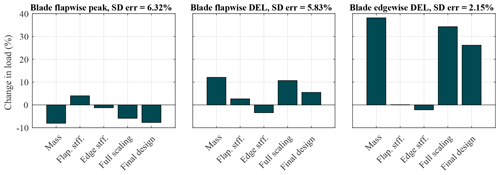Figure 10With η=1.24 and relative to the SUMR-13B with non-scaled structural parameters (${k}_{\mathrm{M}}={k}_{\mathrm{Fs}}={k}_{\mathrm{Es}}=\mathrm{0}$, which yield the SUMR-13B loads in Fig. 9), these plots show the effect of independently scaling the mass (kM=1), flapwise stiffness (kFs=1), and edgewise stiffness (kEs=1), as well as the combined effect of scaling all of the structural parameters (Full Scaling, ${k}_{\mathrm{M}}={k}_{\mathrm{Fs}}={k}_{\mathrm{Es}}=\mathrm{1}$). The standard deviation of the residual is computed using the transformation set in Table 3 and is normalized to the non-scaled SUMR-13B.

### 8.2.1 Selecting kM and kEs for edgewise fatigue loads

The most significant impact of positive structural scaling is the increase in edgewise DELs due to the increased blade mass. Theoretically, the additional mass increase of the larger blade would provide additional reinforcement against these loads, through trailing edge reinforcement or increased root diameter. We see that changes to the blade mass result in a change in edgewise load δmbx, i.e.,

$\begin{array}{}\text{(19)}& \mathit{\delta }{m}_{\mathrm{b}x}={a}_{\mathrm{1}}{k}_{\mathrm{M}}+{b}_{\mathrm{1}},\end{array}$

where a1 and b1 are determined from FAST simulations of the SUMR-13B blade with multiple kM values from 0 to 1 by finding the linear relationship between kM and δmbx. Additional edgewise stiffness must compensate for the increase in edgewise load by increasing the ultimate load:

$\begin{array}{}\text{(20)}& {m}_{\mathrm{ult}}=\frac{\mathrm{2}\mathit{\sigma }{\mathrm{EI}}_{x}}{c},\end{array}$

where σ is the fiberglass strain limit at the trailing edge, EIx is the edgewise stiffness, and c is the blade chord; this is a simplification that assumes the neutral axis is at mid-chord . In terms of the scaling coefficients, a linearized version of Eq. (20) can be obtained:

$\begin{array}{}\text{(21)}& {k}_{\mathrm{Es}}={a}_{\mathrm{2}}\mathit{\delta }{m}_{\mathrm{b}x}+{b}_{\mathrm{2}}.\end{array}$

Finally, changes to the blade structural lay-up in the form of trailing edge reinforcement to increase edgewise stiffness will increase the blade mass:

$\begin{array}{}\text{(22)}& {k}_{\mathrm{M}}={a}_{\mathrm{3}}{k}_{\mathrm{Es}}+{b}_{\mathrm{3}},\end{array}$

where a3 and b3 are determined through a linear regression of SUMR-13B blade designs in NuMAD with a target kEs from 0 to 1. Additional trailing edge reinforcement was applied to meet the target values within 5 % and the kM was computed using the overall mass of the resulting blade model.

The linear system determined by Eqs. (19), (21), and (22) can be solved to determine the necessary structural reinforcement for accommodating the load increase due to the increase in mass. See Table 4 for the results. These parameters can serve as targets for a detailed SUMR-13B structural lay-up design. For the remainder of this study, we will evaluate the loading on other components as a result of the mass increase shown in Table 4.

Table 4Blade structural coefficients for the SUMR-13B blade determined using the relationships described in Fig. 11.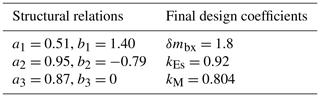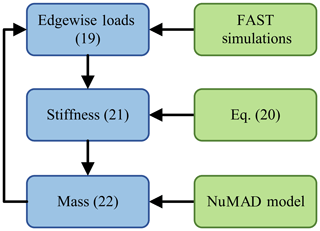Figure 11The relationship between blade mass, edgewise loads, and edgewise stiffness, as well how each value was derived.

9 Hub configuration and main-bearing loads

Blade loads are transferred through the blade root to the hub at the pitch actuator. In this section, we analyze the load cycle amplitudes of the hub loads and how they transfer to the non-rotating turbine components. The hub load axes, yh and zh, rotate with the hub (Fig. 12). About the yh axis, hub loads are directly related to the blade loads for both two- and three-bladed configurations; they peak when the rotor is near ψ=0 due to vertical wind shear, resulting in a large cosine–cyclic component of the hub load about the yh axis (${m}_{\mathrm{h}y,\mathrm{c}}^{\mathrm{1}\mathrm{P}}$). A teeter hinge reduces the coupling between blade and hub loads, except in cases of very large rotor deflections, where “hard” end stops increase the coupling and result in large peak loads. About the zh axis, the source of loading depends on whether the rotor has two or three blades (see Fig. 12). For three-bladed rotors, the hub load about the zh axis is driven by the blade aerodynamic loading due to wind shear and has a similar magnitude to the load about the yh axis (Fig. 12, top right). This symmetry is not inherent in a two-bladed configuration; the mhz load is primarily determined by the weight of the blades unless there is a horizontal wind shear. The mismatch between the load cycle amplitudes of mhy and mhz results in larger non-rotating loads, e.g., msy, for two-bladed rotors (Fig. 12, bottom right). The hub load about the zh axis, for both hub configurations, peaks when the rotor is at ψ=90, resulting in a large ${m}_{\mathrm{h}z,\mathrm{s}}^{\mathrm{1}\mathrm{P}}$ component. The magnitude of these loads in relation to each other is important for determining their impact on the non-rotating load components.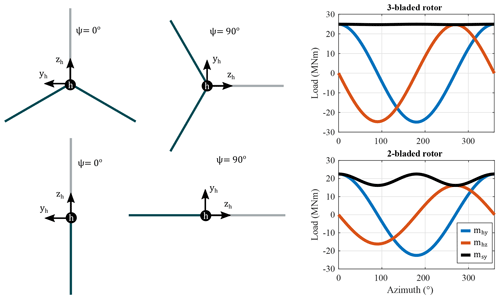Figure 12The hub axis (h) as it rotates with the rotor azimuth angle ψ for a three- and two-bladed rotor. Note that the ys axis in Fig. 5 does not rotate, while the yh axis in Fig. 12 does. An example time series of the hub loads (mhy and mhz) is shown to demonstrate the difference in the non-rotating main-bearing load (msy) for a three-bladed (upper) and two-bladed (lower) SUMR-13B rotor.

The rotating hub is connected to the main shaft, which is supported by a main bearing close to the hub and also may consist of additional bearings between the hub and gearbox. A rotation matrix models the transfer of loads from the rotating to non-rotating frame:

$\begin{array}{}\text{(23)}& \left[\begin{array}{c}{m}_{\mathrm{s}y}\\ {m}_{\mathrm{s}z}\end{array}\right]=\left[\begin{array}{cc}\mathrm{cos}\mathit{\psi }& -\mathrm{sin}\mathit{\psi }\\ \mathrm{sin}\mathit{\psi }& \mathrm{cos}\mathit{\psi }\end{array}\right]\left[\begin{array}{c}{m}_{\mathrm{h}y}\\ {m}_{\mathrm{h}z}\end{array}\right],\end{array}$

which results in the 1P hub loads mapping to large 0P and 2P load components. The large 2P loads result in large fatigue DELs on the non-rotating parts of two-bladed turbines. The hub configuration, including the number of blades, whether a teeter hinge is used, and IPC all have an impact on the fatigue loading of the main bearing.

To compare with the two-bladed SUMR-13B, a three-bladed SUMR-13B was designed using the same blade parameters described in Table 4. Peak and fatigue blade loads in both the flapwise and edgewise directions are unaffected by the change in the number of blades.

Table 5Comparison of the 8.5 ms−1 hub load harmonics for two-bladed fixed, teeter, and IPC methods, as well as three-bladed (3b) rotors, in upwind and downwind positions. We analyze the cosine–cyclic hub load about the yh axis (${m}_{\mathrm{h}y,\mathrm{c}}^{\mathrm{1}\mathrm{P}}$, Fig. 12) and the sine–cyclic hub load about the zh axis (${m}_{\mathrm{h}z,\mathrm{s}}^{\mathrm{1}\mathrm{P}}$) because of their combined effect on non-rotating component loads. The different teeter and IPC methods are presented in Sect. 9.2.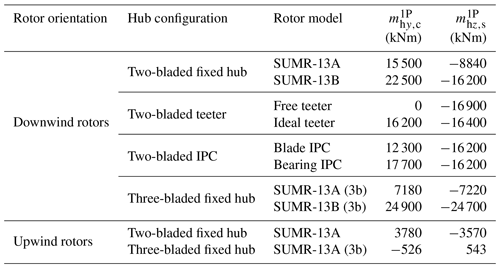Loads on other turbine parts are, however, affected by the change in the number of blades. Hub loads on the two-bladed SUMR-13B are mostly about the yh axis (see ${m}_{\mathrm{h}y,\mathrm{c}}^{\mathrm{1}\mathrm{P}}$ in Table 5), while three-bladed rotors are balanced in both directions. The hub loads in Table 5 can be mapped to the non-rotating frame by Eq. (23). The 1P harmonic in the rotating frame transfers to 0P and 2P harmonics according to

$\begin{array}{}\text{(24)}& & {m}_{\mathrm{s}y}^{\mathrm{0}\mathrm{P}}=\frac{\mathrm{1}}{\mathrm{2}}\left({m}_{\mathrm{h}y,\mathrm{c}}^{\mathrm{1}\mathrm{P}}-{m}_{\mathrm{h}z,\mathrm{s}}^{\mathrm{1}\mathrm{P}}\right)\text{(25)}& & {m}_{\mathrm{s}y}^{\mathrm{2}\mathrm{P}}=\frac{\mathrm{1}}{\mathrm{2}}\left({m}_{\mathrm{h}y,\mathrm{c}}^{\mathrm{1}\mathrm{P}}+{m}_{\mathrm{h}z,\mathrm{s}}^{\mathrm{1}\mathrm{P}}\right).\end{array}$

The 3P component is determined similarly based on the 2P harmonic load components by using Eq. (23).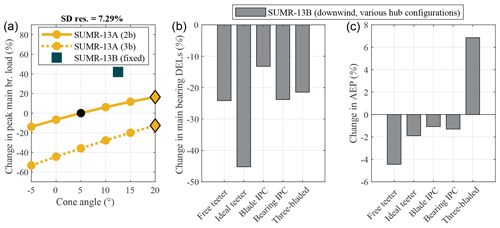Figure 13Change in peak main-bearing loads (a) for the SUMR-13A cone angle study (two- and three-bladed rotors) and the SUMR-13B fixed-hub configuration, change in main-bearing DELs (b) about the ys axis (DELs about the zs axis are within 5 % of the ys-axis DELs) and change in AEP (c) for various hub configurations of the SUMR-13B, compared with the fixed-hub, two-bladed SUMR-13B final design described in Sect. 8.2. The DEL and AEP results from different hub configurations (b, c) are design loads computed directly from DLC simulations.

## 9.2 Teeter and individual pitch control

Historically, some two-bladed turbines have used a mechanical teeter hinge, which allows for rotation about an axis perpendicular to the main shaft at the shaft tip. Recently, with the advent of pitch regulated turbines, individual pitch controllers have been designed in order to mimic this action by changing the aerodynamic loads on the blades as they rotate. Both solutions reduce loading on the hub, which translates into reduced loading on the main bearing and other non-rotating components.

We have modeled a free-teetering hinge in FAST by enabling the teeter degree-of-freedom and setting a zero damping coefficient to the teeter motion. This free-teetering setup would provide the best configuration for reducing blade loads. A more realistic teeter hinge must account for friction, damping, and end stops (see, e.g., ).

The free-teetering hinge configuration completely eliminates the coupling between blade and hub loads, resulting in zero hub loads about the yh axis. The relationship in Eq. (25) and harmonic loads in Table 5 suggest that main-bearing fatigue loads (${m}_{\mathrm{s}y}^{\mathrm{2}\mathrm{P}}$) increase when compared to the fixed-hub configuration. However, DLC simulations show that turbulence has a relatively minimal impact on the non-rotating components for this rotor with a free-teetering hinge, compared with all other rotors. In other words, the design loads for the main bearing are nearly equal to the harmonic loads, but in every other case there is a significant turbulent component, as mentioned in Sect. 6. Since this case is an outlier and behaves differently when mapping harmonic loads to turbulent loads, it is omitted from the transformation set of two-bladed rotors. Instead of presenting the transformed load estimates and power capture, we present the design loads computed directly from DLC simulations in Fig. 13. However, the harmonic loads in Table 5 still illustrate how an optimal teeter design could mimic the balanced hub loads of three-bladed rotors.

A more ideal teeter design could be achieved by selecting an appropriate teeter damping coefficient dteet that matches the ${m}_{\mathrm{h}y,\mathrm{c}}^{\mathrm{1}\mathrm{P}}$ and ${m}_{\mathrm{h}z,\mathrm{s}}^{\mathrm{1}\mathrm{P}}$ load harmonics to minimize the main-bearing load ${m}_{\mathrm{s}y}^{\mathrm{2}\mathrm{P}}$. Since only one damping coefficient must be designed for all wind speeds, we minimize the main-bearing load using the wind speed distribution p(u) by

$\begin{array}{}\text{(26)}& {d}_{\mathrm{teet},\mathrm{opt}}={\mathrm{argmin}}_{{d}_{\mathrm{teet}}}\sum _{u\in {U}_{\mathrm{teet}}}p\left(u\right){m}_{\mathrm{s}y}^{\mathrm{2}\mathrm{P}},\end{array}$

where Uteet is the set of wind speeds used to analyze the teeter damping, focused on below-rated operation, where the greatest fatigue contribution occurs. Main-bearing load cycle amplitudes (${m}_{\mathrm{s}y}^{\mathrm{2}\mathrm{P}}$ and ${m}_{\mathrm{s}z}^{\mathrm{2}\mathrm{P}}$) increase with wind speed due to the increased effect of wind shear, but lower wind speeds are far more probable than high wind speeds. Since our design goal is to reduce fatigue loads on the main bearing and other non-rotating components, we focus on below-rated wind conditions. The ideal teeter design greatly reduces the main-bearing fatigue loads, along with the fatigue loading on the other non-rotating components but reduces energy capture by 1.9 %, compared with the fixed two-bladed SUMR-13B (Fig. 13b, c).

Alternatively, IPC can be used to mimic the rotor balancing of a teeter hinge by adding a time-varying pitch angle offset to each blade. An IPC algorithm was initially designed to focus on blade loads, which we call blade IPC in Table 5 and Fig. 13. The two-bladed IPC architecture used here was initially presented in , which minimizes the teeter load:

$\begin{array}{}\text{(27)}& {m}_{\mathrm{teet}}=\frac{\mathrm{1}}{\mathrm{2}}\left({m}_{\mathrm{b}y,\mathrm{1}}-{m}_{\mathrm{b}y,\mathrm{2}}\right).\end{array}$

Using the relationship in Eq. (25), we designed a bearing IPC algorithm with the goal of balancing the hub load components, such that ${m}_{\mathrm{h}y,\mathrm{c}}^{\mathrm{1}\mathrm{P}}=-{m}_{\mathrm{h}z,\mathrm{s}}^{\mathrm{1}\mathrm{P}}$, to minimize 2P loading on the main bearing. Equivalently, ${m}_{\mathrm{h}y}^{\mathrm{1}\mathrm{P}}$ and ${m}_{\mathrm{h}z}^{\mathrm{1}\mathrm{P}}$ should be equal in magnitude and 90 out of phase. Since $|{m}_{\mathrm{h}z}^{\mathrm{1}\mathrm{P}}|$ changes more slowly than $|{m}_{\mathrm{h}y}^{\mathrm{1}\mathrm{P}}|$, the mhz signal is delayed by 90 and the difference,

$\begin{array}{}\text{(28)}& {m}_{\mathrm{d}}={m}_{\mathrm{h}y}-{m}_{\mathrm{h}z}\left(\mathit{\psi }-\mathrm{90}{}^{\circ }\right),\end{array}$

can be fed back using the same architecture as the blade IPC because mhy=2mteet. Harmonic load estimates suggest better load mitigation than those in Fig. 13, so we present the DLC-based design loads directly from turbulent simulations. In general, dynamic control solutions are not as well estimated using harmonic load estimates, compared with changes to the rotor model using the same control because dynamics due to turbulence often drive control design. Other control methods were attempted to balance the load components in Eq. (25), which are further explored in .

If used in below-rated conditions, these load mitigation techniques reduce power capture, as shown in Fig. 13c. IPC can be designed so that it only operates in above-rated conditions, resulting in a negligible power loss. However, this reduces its effectiveness in constraining peak loads that occur close to rated wind speeds.

## 9.3 Large cone angle effects

The main bearing must support the weight of the rotor and thrust imbalance on the rotor due to shear, i.e.,

$\begin{array}{}\text{(29)}& {m}_{\mathrm{s}y}^{\mathrm{0}}={m}_{\mathrm{s}y,\mathrm{grav}}^{\mathrm{0}}+{m}_{\mathrm{s}y,\mathrm{shr}}^{\mathrm{0}}.\end{array}$

For downwind turbines, both components of Eq. (29) are positive, resulting in large, constant main-bearing loads about the ys axis. For upwind turbines, the load due to gravity ${m}_{\mathrm{s}y,\mathrm{grav}}^{\mathrm{0}}$ is negative, while the load due to wind shear ${m}_{\mathrm{s}y,\mathrm{shr}}^{\mathrm{0}}$ is positive, which greatly reduces the steady-state main-bearing load for upwind turbines compared to downwind turbines. To quantify this difference, we analyze the harmonic load estimate of the peak main bearing (${m}_{\mathrm{s}y,\mathrm{Peak}}={m}_{\mathrm{s}y}^{\mathrm{0}\mathrm{P}}+{m}_{\mathrm{s}y}^{\mathrm{2}\mathrm{P}}$) for rotors with various cone angles (Fig. 13a).

The harmonic loads in Table 5 suggest there would be a significant change in the mean main-bearing load ${m}_{\mathrm{s}y}^{\mathrm{0}\mathrm{P}}$ going from upwind to downwind rotor configurations. However, the design loads computed using DLC simulations show that turbulence contributes a large amount to the peak load experienced by the main bearing (Fig. 6) for both configurations. A downwind configuration, compared to the same rotor upwind (with cone angles of ±5, respectively) only increases the main-bearing load by about 15 %. Despite the larger total blade mass of the three-bladed rotors, two-bladed rotors still have a larger peak load due to the increased 2P loading and a larger turbulent load component. We see this same effect in the fatigue loading results of Fig. 13, which suggests that peak main-bearing loads could be reduced using the same methods as in Sect. 9.2. The larger SUMR-13B, however, has a non-negligible increase in the peak main-bearing load, due to combined increases in blade mass, blade length, and cone angle. These increased loads on the main-bearing transfer to the other non-rotating components, which we will analyze in the yaw-bearing and tower design studies.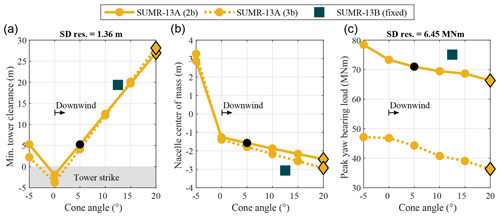Figure 14The tower clearance (a) resulting from upwind (negative cone angles) and downwind (positive cone angles) configurations, the nacelle center of mass (b) required to balance the rotors, and the peak yaw-bearing loads (c) of the balanced rotors.

10 Yaw-bearing loads and nacelle layout

The main bearing is mounted to the bedplate of the nacelle, which attaches to the yaw bearing, responsible for rotating the entire nacelle and rotor to align with the wind direction. The yaw bearing experiences similar loads to the main bearing; they peak near rated and at cut-out due to thrust effects and wind shear, respectively. A potential issue with downwind turbines is a large, mean yy-axis moment leading to large peak yaw-bearing loads, similar to the peak main-bearing load. However, peak loads on the yaw bearing can be counteracted by properly balancing the nacelle center of mass atop the tower. We will study the different cone angle designs from Sect. 8.1 for two- and three-bladed rotors, as well as our SUMR-13B final design to investigate the effect of rotor cone angle and increased mass on nacelle design and yaw-bearing loads.

Large mean loads on the yaw bearing (${m}_{\mathrm{y}y}^{\mathrm{0}\mathrm{P}}$) cause large peak loads that can be overcome by properly choosing the hub-to-tower overhang xOH and the nacelle center of mass xcm (as shown in Fig. 5). We use a simple method for determining the nacelle overhang: for upwind turbines, the nacelle overhang was set to that of the CONR-13 (−8.61 m), and for downwind turbines, we used the minimum possible overhang (3.15 m, equal to the radius of the tower at the nacelle). These hub-to-tower overhang values result in adequate tower clearance (the minimum perpendicular distance between the blade tip and the yaw axis yz) when the cone angle is at least 5 away from the tower (Fig. 14a). However, such an important design parameter would certainly be subject to verification using a detailed tower design and the full set of DLCs before deeming the tower safe from blade strike. Rotors with larger cone angles have large tower clearances, which is part of the motivation for their design.

To compare peak yaw-bearing loads across rotors, we adjust the nacelle center of mass so that mean yaw-bearing loads (${m}_{\mathrm{y}y}^{\mathrm{0}\mathrm{P}}$) are minimized in still air. The mean yaw-bearing load is linearly dependent on the component masses and center of masses:

$\begin{array}{}\text{(30)}& {m}_{\mathrm{y}y}^{\mathrm{0}\mathrm{P}}=g\left({m}_{\mathrm{nac}}{x}_{\mathrm{cm}}+{m}_{\mathrm{rot}}{x}_{\mathrm{cm},\mathrm{rot}}\right),\end{array}$

where g is the acceleration due to gravity, mnac is the nacelle mass, mrot is the total rotor mass, and xcm,rot is the rotor center of mass. The nacelle center of mass xcm that sets the mean overturning yaw-bearing load to zero is

$\begin{array}{}\text{(31)}& {x}_{\mathrm{cm}}=-\frac{{m}_{\mathrm{rot}}{x}_{\mathrm{cm},\mathrm{rot}}}{{m}_{\mathrm{nac}}}.\end{array}$

The hub and nacelle masses are approximated using a length-to-mass scaling factor of ${\left(\frac{\mathrm{100}}{\mathrm{63}}\right)}^{\mathrm{3}}$ from the NREL 5 MW reference turbine and shown in Table 6. The hub and nacelle masses are constant for all rotors throughout this study, but the rotor mass and center of mass vary.

Table 6Component masses for placing the nacelle center of mass atop the tower.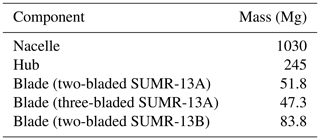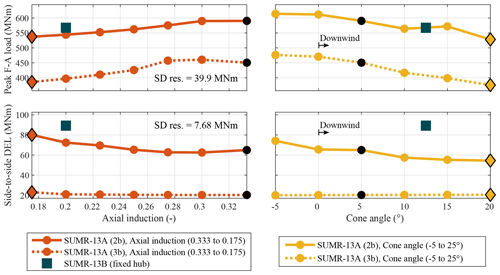Figure 15Peak tower loads in the fore–aft (F-A) direction (${m}_{\mathrm{t}y}^{\mathrm{Peak}}$) and side-to-side DELs (${m}_{\mathrm{t}x}^{\mathrm{DEL}}$) for rotors with different axial induction factors (and corresponding blade length changes as discussed in Sect. 8.1; red), cone angles (yellow), and number of blades. The same loads for the SUMR-13B are also shown. Unless otherwise specified, the available rotor power is 13.9 MW, the axial induction is 0.333, and the cone angle is 5; the SUMR-13B is specified in Table 1. The standard deviation of the residual for both load axes incorporates all of the presented design studies.

Rotors with large downwind cone angles must have nacelle center of masses further upwind (negative values in Fig. 14, center). Given the nacelle mass in Table 6, moving the nacelle center of mass 1 m upwind reduces the mean (and peak) yaw moment by about 10 MNm. Due to the extra overhang necessary for upwind turbines, the center of mass location for the downwind turbines is closer to the tower than for the upwind turbines. By designing the proper hub-to-tower overhang and nacelle placement, the peak yaw loads are no more problematic for downwind rotors than upwind rotors. Once properly balanced, the peak yaw loads are primarily driven by the thrust imbalance due to wind shear, which decreases with increased cone angle (Fig. 14c). However, changing the nacelle center of mass is a non-trivial task that involves a detailed drivetrain and nacelle design. Fatigue loads (not shown) on the yaw bearing also depend on rotor thrust and decrease with increasing cone angles. The methods presented in Sect. 9.2 also reduce yaw-bearing loads.

The yaw bearing is attached to the top of the tower, which must support the rotor–nacelle assembly and withstand large moments. We focus on the effect of rotor axial induction, cone angle, and the number of blades on peak loads in the fore–aft direction ${m}_{\mathrm{t}y}^{\mathrm{Peak}}$ and fatigue loading in the side-to-side direction ${m}_{\mathrm{t}x}^{\mathrm{DEL}}$.

Besides having larger chord lengths that sample more turbulence than three-bladed rotors, two-bladed rotors also experience a resonance due to the tower design. Modern wind turbine towers are usually designed to be “soft–stiff”, with a natural frequency between the 1P and 3P harmonics of the rotor . When the 2P rotor speed interacts with the natural frequency of the tower, there are high fore–aft and side-to-side loads. Side-to-side tower DELs increase the most, since there is less aerodynamic damping from the rotor in this direction . One idea is to use a high-compliance tower structure or a floating substructure with a natural frequency below the 1P harmonic. However, a very low tower natural frequency causes tower motion to be perceived as a wind speed disturbance, resulting in speed regulation issues. Several studies have considered this, given the emergence of floating wind turbines , but to simplify our analysis, we have kept the same tower for all turbines: a scaled version of the NREL 5 MW three-bladed reference model .

The harmonic load simulations predict the same peak tower loads for both two- and three-bladed rotors, but turbulent simulations show a clear difference in the design load, as indicated in Fig. 15. Compared with other turbine parts, the transformed estimates of the tower loads have a large amount of uncertainty (Fig. 6). This uncertainty can be attributed to the source of these tower loads, which are highly dependent on turbulent gusts.

12 Model limitations, suggested improvements, and potential use

When analyzing the design studies of Sects. 811, we have come across a few sources of uncertainty in the estimates of the transformed loads. When mapping the harmonic loads to the loads calculated using DLCs (Sect. 6), we see that a large component of the design load is due to turbulence, which primarily depends on the number of blades on the rotor, leading to different transformation coefficients for two- and three-bladed rotors in Eq. (14). However, the turbulent component is also correlated with other model parameters, most notably rotor thrust. Highly coned downwind rotors reduce the rotor thrust and have a lower turbulent component than upwind rotors. Different levels of turbulence, besides Class IIB that was analyzed in this study, would result in different turbulent components and residuals of the transformation from harmonic to design load. Additionally, dynamic effects, like the problematic gust in Fig. 3, are not explicitly modeled in the harmonic model of Sect. 5. Thus, dynamic control solutions that appear promising in constant wind inputs should be ultimately verified in turbulent simulations.

Several improvements to the harmonic model could be made. For instance, the problematic gust events follow a similar profile in many instances; this could be an additional simulation added to the model's set of simulations. While outside the scope of this study, parked, fault, and shutdown cases can result in the largest design loads in practice, e.g., in ; they could be added with little computational expense. The transformation procedure could be streamlined by perhaps doing a single, exemplary turbulent simulation for each case to determine the turbulent component of each load.

The harmonic loads and their mapping to design load estimates used to evaluate design trade-offs provide a potential middle ground for wind turbine system engineering tools. The method is more realistic than simple scaling rules and static estimates but requires less computational effort than full sets of DLC simulations and therefore allows for an initial optimization over a wider range of configurations.

13 Conclusions

• Low axial induction rotors using longer blades with smaller chord lengths can capture more energy while constraining peak operational blade loads.

• As rotor size increases, due to increasing blade mass, edgewise blade loading becomes a critical design-driving load and may ultimately constrain the size of wind turbine rotors.

• Downwind, coned rotors can significantly reduce peak operational blade loads but capture less energy than rotors with lower cone angles.

• Downwind, coned rotors will experience slightly larger (about 15 %–25 %) peak main-bearing loads than upwind turbines, but the effect is amplified with increasing blade length, mass, and cone angle.

• Peak yaw-bearing and tower loads are not problematic for downwind rotors as long as the nacelle is properly balanced on the tower.

• Two-bladed rotors experience significantly greater loading on the non-rotating parts compared to three-bladed rotors, unless a teeter hinge or individual pitch control is utilized. In these cases, the loading is comparable but with a loss in power.

• Two-bladed rotors will require either speed avoidance control or a different tower design to avoid resonance with the 2P frequency of the rotor.

We believe that our model has provided future wind turbine designers with a method for more quickly analyzing design trade-offs, and our design studies can serve as a reference for future large rotor designs.

Code and data availability
Code and data availability.

The code and/or data from this study can be made available upon request.

Author contributions
Author contributions.

All authors contributed to the baseline models and design direction of the SUMR rotors. DSZ developed the harmonic model, transformation, and closed-loop controllers, carried out simulations, and prepared the visualizations and manuscript. GKA designed the aerodynamic properties of the various rotors, MC investigated the structural properties, DPM investigated the teeter configurations, and CJB visualized the design studies. KEJ provided a thorough review of initial and the final drafts. EL developed the original rotor concept and outlined system-level goals. DTG provided experience on edgewise loading for large rotors, guided the structural design process, and reviewed the article. MSS reviewed the article. LYP had a supervising function and guided the study, helped formulate the article concept, and reviewed multiple drafts of the article.

Competing interests
Competing interests.

The authors declare that they have no conflict of interest.

Acknowledgements
Acknowledgements.

The information, data, or work presented herein was funded in part by the Advanced Research Projects Agency – Energy (ARPA-E), US Department of Energy, under award no. DE-AR0000667. The views and opinions of authors expressed herein do not necessarily state or reflect those of the United States Government or any agency thereof. Support from the Hanse-Wissenschaftskolleg Institute for Advanced Study (Delmenhorst, Germany) and a Palmer Endowed Chair Professorship are also gratefully acknowledged. The authors would also like to acknowledge Paul Veers for his helpful review of this article on behalf of the National Renewable Energy Laboratory and the entire SUMR team for the discussions that ultimately motivated these design studies, as well as their work on the many design aspects of the baseline rotor models.

Financial support
Financial support.

This research has been supported by the Advanced Research Projects Agency – Energy (grant no. DE-AR0000667).

Review statement
Review statement.

This paper was edited by Raúl Bayoán Cal and reviewed by Christopher Kelley and one anonymous referee.

References

Ananda, G. K., Bansal, S., and Selig, M. S.: Aerodynamic design of the 13.2 MW SUMR-13i wind turbine rotor, in: 2018 Wind Energy Symposium, AIAA SciTech Forum (AIAA 2018-0994), available at: https://arc.aiaa.org/doi/10.2514/6.2018-0994 (last access: 30 October 2019), 2018. a

Bak, C., Zahle, F., Bitsche, R., Kim, T., Yde, A., Henriksen, L. C., Natarajan, A., and Hansen, M. H.: Description of the DTU 10-MW reference wind turbine, Tech. Rep. I-0092, DTU Wind Energy, available at: https://orbit.dtu.dk/files/55645274/The_DTU_10MW_Reference_Turbine_Christian_Bak.pdf (last access: 30 October 2019), 2013. a

Berg, J. and Resor, B.: Numerical manufacturing and design tool (NuMAD V2.0) for wind turbine blades: user's guide, Tech. Rep. SAND2012-728, Sandia National Laboratories, available at: https://energy.sandia.gov/wp-content/gallery/uploads/NuMAD_UserGuide_SAND2012-7028.pdf (last access: 30 October 2019), 2012. a

Bergami, L., Madsen, H. A., and Rasmussen, F.: A two-bladed teetering hub configuration for the DTU 10 MW RWT: loads considerations, in: European Wind Energy Association (EWEA), 1–8, available at: http://orbit.dtu.dk/files/89872770/prod11395144001651.leob_Ewea2Bl_ver2.pdf (last access: 30 October 2019), 2014. a, b, c

Bertelè, M., Bottasso, C. L., Cacciola, S., Daher Adegas, F., and Delport, S.: Wind inflow observation from load harmonics, Wind Energ. Sci., 2, 615–640, https://doi.org/10.5194/wes-2-615-2017, 2017. a

Bortolotti, P., Bottasso, C. L., and Croce, A.: Combined preliminary-detailed design of wind turbines, Wind Energ. Sci., 1, 71–88, https://doi.org/10.5194/wes-1-71-2016, 2016. a, b

Bottasso, C. L. and Cacciola, S.: Model-independent periodic stability analysis of wind turbines, Wind Energy, 18, 865–887, https://doi.org/10.1002/we.1735, 2015. a

Bottasso, C. L., Croce, A., Riboldi, C. E., and Nam, Y.: Multi-layer control architecture for the reduction of deterministic and non-deterministic loads on wind turbines, Renew. Energy, 51, 159–169, https://doi.org/10.1016/j.renene.2012.08.079, 2013. a

Budynas, R. G. and Nisbett, J. K.: Shigley's Mechanical Engineering Design, 9th Edn., McGraw-Hill, New York, NY, available at: https://eclass.teicrete.gr/modules/document/file.php/TM114/shigley-machine-design-.pdf (last access: 30 October 2019), 2015. a

Dimitrov, N., Kelly, M. C., Vignaroli, A., and Berg, J.: From wind to loads: wind turbine site-specific load estimation with surrogate models trained on high-fidelity load databases, Wind Energ. Sci., 3, 767–790, https://doi.org/10.5194/wes-3-767-2018, 2018. a

Døssing, M.: Optimization of wind turbine rotors – using advanced aerodynamic and aeroelastic models and numerical optimization, Tech. Rep. Risø-PhD No. 69, Technical University of Denmark, available at: https://www.osti.gov/etdeweb/servlets/purl/1021046 (last access: 30 October 2019), 2011. a

Drela, M.: XFOIL: an analysis and design system for low Reynolds number airfoils, in: Low Reynolds Number Aerodynamics, edited by: Mueller, T. J., Springer, Berlin, Heidelberg, 1–12, 1989. a

Drela, M. and Giles, M.: Viscous-inviscid analysis of transonic and low Reynolds number airfoils, AIAA J., 25, 1347–1355, https://doi.org/10.2514/3.9789, 1987. a

Dykes, K., Ning, A., King, R., Graf, P., Scott, G., and Veers, P.: Sensitivity analysis of wind plant performance to key turbine design parameters: a systems engineering approach, Tech. Rep. NREL/CP-5000-60920, National Renewable Energy Laboratory, available at: https://www.nrel.gov/docs/fy14osti/60920.pdf (last access: 30 October 2019), 2014. a

Griffith, D. T.: The SNL100-01 blade: carbon design studies for the Sandia 100-meter blade, Tech. Rep. SAND2013-1178, Sandia National Laboratory, available at: http://prod.sandia.gov/techlib/access-control.cgi/2013/131178.pdf (last access: 30 October 2019), 2013a. a

Griffith, D. T.: The SNL100-02 blade: advanced core material design studies for the Sandia 100-meter blade, Tech. Rep. SAND2013-10162, Sandia National Laboratory, available at: http://energy.sandia.gov/wp-content/gallery/uploads/dlm_uploads/1310162.pdf (last access: 30 October 2019), 2013b. a

Griffith, D. T.: Structural design of the SUMR-13 wind turbine blade, Tech. Rep. M2.5.9, Advanced Research Projects Agency – Energy (ARPA-E), Segmented Ultralight Morphing Rotor (SUMR), available at: https://arpa-e.energy.gov/?q=slick-sheet-project/ultra-large-wind-turbine (last access: 30 October 2019), 2017. a, b

Griffith, D. T. and Ashwill, T. D.: The Sandia 100-meter all-glass baseline wind turbine blade: SNL100-00, Tech. Rep. SAND2011-3779, Sandia National Laboratory, available at: https://energy.sandia.gov/wp-content/gallery/uploads/113779.pdf (last access: 30 October 2019), 2011. a, b

Griffith, D. T. and Richards, P. W.: The SNL100-03 blade: design studies with flatback airfoils for the Sandia 100-meter blade, Tech. Rep. SAND2014-18129, Sandia National Laboratory, available at: http://energy.sandia.gov/wp-content/gallery/uploads/dlm_uploads/1418129.pdf (last access: 30 October 2019), 2014. a, b, c

Hayman, G. J.: MLife theory manual for version 1.00, Tech. rep., National Renewable Energy Laboratory, available at: https://nwtc.nrel.gov/system/files/MLife_Theory.pdf (last access: 30 October 2019), 2012. a, b

Ichter, B., Steele, A., Loth, E., Moriarty, P., and Selig, M.: A morphing downwind-aligned rotor concept based on a 13-MW wind turbine, Wind Energy, 19, 625–637, https://doi.org/10.1002/we.1855, 2016. a

International Electrotechnical Commission: Wind turbines – part 1: design requirements, Tech. Rep. IEC 61400-1:2005(E), available at: https://www.saiglobal.com/pdftemp/previews/osh/iec/iec61000/61400/iec61400-1\%7Bed3.0\%7Den.pdf (last access: 30 October 2019), 2005. a, b, c

Jenkins, N., Burton, A., Sharpe, D., and Bossanyi, E.: Wind Energy Handbook, John Wiley & Sons Ltd, UK, 2001. a

Johnson, K., Fingersh, L. J., and Wright, A. D.: Controls advanced research turbine: lessons learned during advanced controls testing, Tech. Rep. NREL/TP-500-38130, National Renewable Energy Laboratory, available at: https://pdfs.semanticscholar.org/6f07/7588aca0278bc87e6f2b9dba5e4492960d44.pdf (last access: 30 October 2019), 2005. a

Jonkman, B. and Kilcher, L.: TurbSim user's guide: version 1.06.00, Tech. Rep. TP-500-39797, National Renewable Energy Laboratory, available at: https://nwtc.nrel.gov/system/files/TurbSim.pdf (last access: 30 October 2019), 2012. a

Jonkman, J. M.: The new modularization framework for the FAST wind turbine CAE tool, in: 51st AIAA Aerospace Sciences Meeting, available at: https://www.nrel.gov/docs/fy13osti/57228.pdf (last access: 30 October 2019), 2013. a

Jonkman, J. M. and Matha, D.: Dynamics of offshore floating wind turbines - analysis of three concepts, Wind Energy, 14, 557–569, https://doi.org/10.1002/we.442, 2011. a, b

Jonkman, J. M., Butterfield, S., Musial, W., and Scott, G.: Definition of a 5-MW reference wind turbine for offshore system development, Tech. Rep. NREL/TP-500-38060, National Renewable Energy Laboratory, available at: https://www.nrel.gov/docs/fy09osti/38060.pdf (last access: 30 October 2019), 2009. a, b, c, d

Loth, E., Fingersh, L., Griffith, D., Kaminski, M., and Qin, C.: Gravo-aeroelastically scaling for extreme-scale wind turbines, in: 35th AIAA Applied Aerodynamics Conference, 5–9 June 2017, Denver, Colorado, 1–11, https://doi.org/10.2514/6.2017-4215, 2017a. a

Loth, E., Steele, A., Qin, C., Ichter, B., Selig, M. S., and Moriarty, P.: Downwind pre-aligned rotors for extreme-scale wind turbines, Wind Energy, 20, 1241–1259, https://doi.org/10.1002/we.2092, 2017b. a

McFarlane, D. and Glover, K.: A loop-shaping design procedure using H synthesis, IEEE T. Automat. Control, 37, 759–769, https://doi.org/10.1109/9.256330, 1992. a

McWilliam, M. K., Barlas, T. K., Madsen, H. A., and Zahle, F.: Aero-elastic wind turbine design with active flaps for AEP maximization, Wind Energ. Sci., 3, 231–241, https://doi.org/10.5194/wes-3-231-2018, 2018. a

Mone, C., Hand, M., Bolinger, M., Rand, J., Heimiller, D., and Ho, J.: 2015 cost of wind energy review, Tech. Rep. NREL/TP-6A20-66861, National Renewable Energy Laboratory, available at: https://www.nrel.gov/docs/fy17osti/66861.pdf (last access: 30 October 2019), 2015. a, b

Natarajan, A., Hansen, M. H., and Wang, S.: Design load basis for offshore wind turbines, Tech. Rep. E-0133, DTU Wind Energy, available at: https://orbit.dtu.dk/files/126478218/DTU_Offshore_Design_Load_Basis_Rev_0.pdf (last access: 30 October 2019), 2016. a

Ning, A., Damiani, R., and Moriarty, P. J.: Objectives and constraints for wind turbine optimization, J. Solar Energ. Eng., 136, 041010, https://doi.org/10.1115/1.4027693, 2014. a

Noyes, C., Qin, C., Loth, E., and Schreck, S.: Measurements and predictions of wind turbine tower shadow and fairing effects, J. Wind Eng. Indust. Aerodynam., 179, 297–307, https://doi.org/10.1016/j.jweia.2018.06.012, 2018. a

Pao, L. Y. and Johnson, K. E.: Control of wind turbines, IEEE Control Syst. Mag., 31, 44–62, https://doi.org/10.1109/MCS.2010.939962, 2011. a

Pavese, C., Tibaldi, C., Larsen, T. J., Kim, T., and Thomsen, K.: Reduced design load basis for ultimate blade loads estimation in multidisciplinary design optimization frameworks, J. Phys.: Conf. Ser., 753, 62005, https://doi.org/10.1088/1742-6596/753/6/062005, 2016. a

Pavese, C., Tibaldi, C., Zahle, F., and Kim, T.: Aeroelastic multidisciplinary design optimization of a swept wind turbine blade, Wind Energy, 20, 1941–1953, https://doi.org/10.1002/we.2131, 2017. a, b

Peeringa, J., Brood, R., Ceyhan, O., Engels, W., and de Winkel, G.: Upwind 20 MW wind turbine pre-design: blade design and control, Tech. Rep. ECN-E–11-017, Energy Research Centre of the Netherlands, available at: https://www.ecn.nl/publicaties/PdfFetch.aspx?nr=ECN-E–11-017 (last access: 30 October 2019), 2011. a

Phillips, C. L., Parr, J., and Riskin, E.: Signals, Systems, and Transforms, 4th Edn., Prentice Hall Press, Upper Saddle River, NJ, USA, 2007. a

Robertson, A., Sethuraman, L., Jonkman, J., and Quick, J.: Assessment of wind parameter sensitivity on ultimate and fatigue wind turbine loads: preprint, Tech. Rep. NREL/CP-5000-70445, National Renewable Energy Laboratory, available at: https://www.nrel.gov/docs/fy18osti/70445.pdf (last access: 30 October 2019), 2018. a

Schorbach, V., Dalhoff, P., and Gust, P.: Teeter design for lowest extreme loads during end impacts, Wind Energy, 21, 1–14, https://doi.org/10.1002/we.2140, 2017. a

Selig, M.: PROPID – software for horizontal-axis wind turbine design and analysis, available at: http://www.ae.illinois.edu/m-selig/propid.html (last access: 30 October 2019), 1995. a

Selig, M. and Tangler, J.: Development and application of a multipoint inverse design method for horizontal axis wind turbines, Wind Eng., 19, 91–105, 1995. a

Sieros, G., Chaviaropoulos, P., Sørensen, J. D., Bulder, B. H., and Jamieson, P.: Upscaling wind turbines: theoretical and practical aspects and their impact on the cost of energy, Wind Energy, 15, 3–17, https://doi.org/10.1002/we.527, 2012. a

Tibaldi, C., Hansen, M. H., and Zahle, F.: Methods for systematic tuning of wind turbine controllers, Tech. Rep. E-0100, DTU Wind Energy, available at: http://orbit.dtu.dk/files/118777390/Wind_Energy_E_0100.pdf (last access: 30 October 2019), 2015. a

van der Tempel, J. and Molenaar, D. P.: Wind turbine structural dynamics – a review of the principles for modern power generation, onshore and offshore, Wind Eng., 26, 211–222, https://doi.org/10.1260/030952402321039412, 2003. a

van Solingen, E. and van Wingerden, J. W.: Linear individual pitch control design for two-bladed wind turbines, Wind Energy, 18, 677–697, https://doi.org/10.1002/we.1720, 2015. a

Zahle, F., Tibaldi, C., Verelst, D. R., Bak, C., Bitsche, R., and Blasques, J. P.: Aero-elastic optimization of a 10 MW wind turbine, in: 33rd Wind Energy Symposium, AIAA SciTech Forum (AIAA 2015-0491), 5–9 January 2015, Kissimmee, Florida, https://doi.org/10.2514/6.2015-0491, 2015.  a

Zalkind, D. S. and Pao, L. Y.: A harmonic model for loads analysis and control design of a 2-bladed wind turbine, in: 2019 AIAA SciTech Forum and Exposition, available at: https://sumrsite.files.wordpress.com/2018/08/harmonic-model-loads.pdf, last access: 30 October 2019. a

Zalkind, D. S., Pao, L. Y., Martin, D. P., and Johnson, K. E.: Models used for the simulation and control of a segmented ultralight morphing rotor, in: 20th IFAC World Congress, 10–14 July 2017, Toulouse, France, 4564–4569, https://doi.org/10.1016/j.ifacol.2017.08.377, 2017. a

The available rotor power of 13.9 MW at 11.3 ms−1 and rated generator power of 13.2 MW correspond to a 95 % generator efficiency.